# Set Builder Notation

Set Builder Notation

We see objects all around us. Some of them are grouped together and some form a collection of objects.

A set represents a collection of things, objects, or numbers.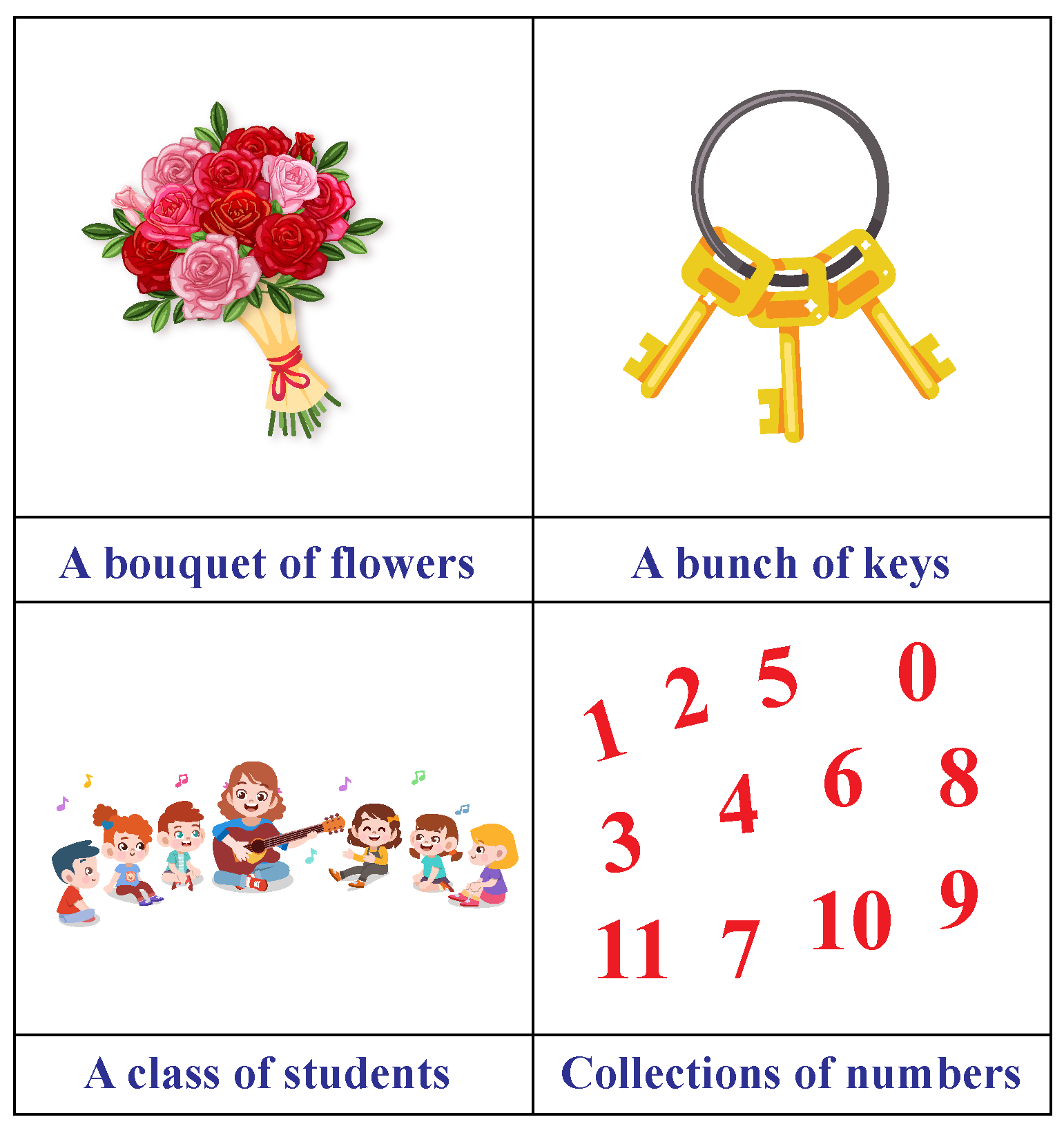We have special words to indicate each of the collection of objects shown above.

In all the above examples we can clearly specify the objects of that collection.

Such a collection of defined objects is termed a "Set."

## Lesson Plan

 1 What Is Set Builder Notation? 2 Challenging Questions on Set Builder Notations 3 Important Notes on Set Builder Notations 4 Solved Examples on Set Builder Notations 5 Interactive Questions on Set Builder Notations

## What Is a Set?

### Set

If we can clearly define and decide the objects of a given collection, then that collection is called a set.

We typically use capital letters to name the set A, B, C,....., and so on.

The contents or the elements of the set are denoted by using lower-case letters a, b, c,..., and so on.

If $$a$$ is an element of set $$A$$, then we write it as ‘$$a \in A$$’ and if $$a$$ is not an element of set $$A$$ then we write $$a \not \in A$$.

The symbols for number sets are presented below.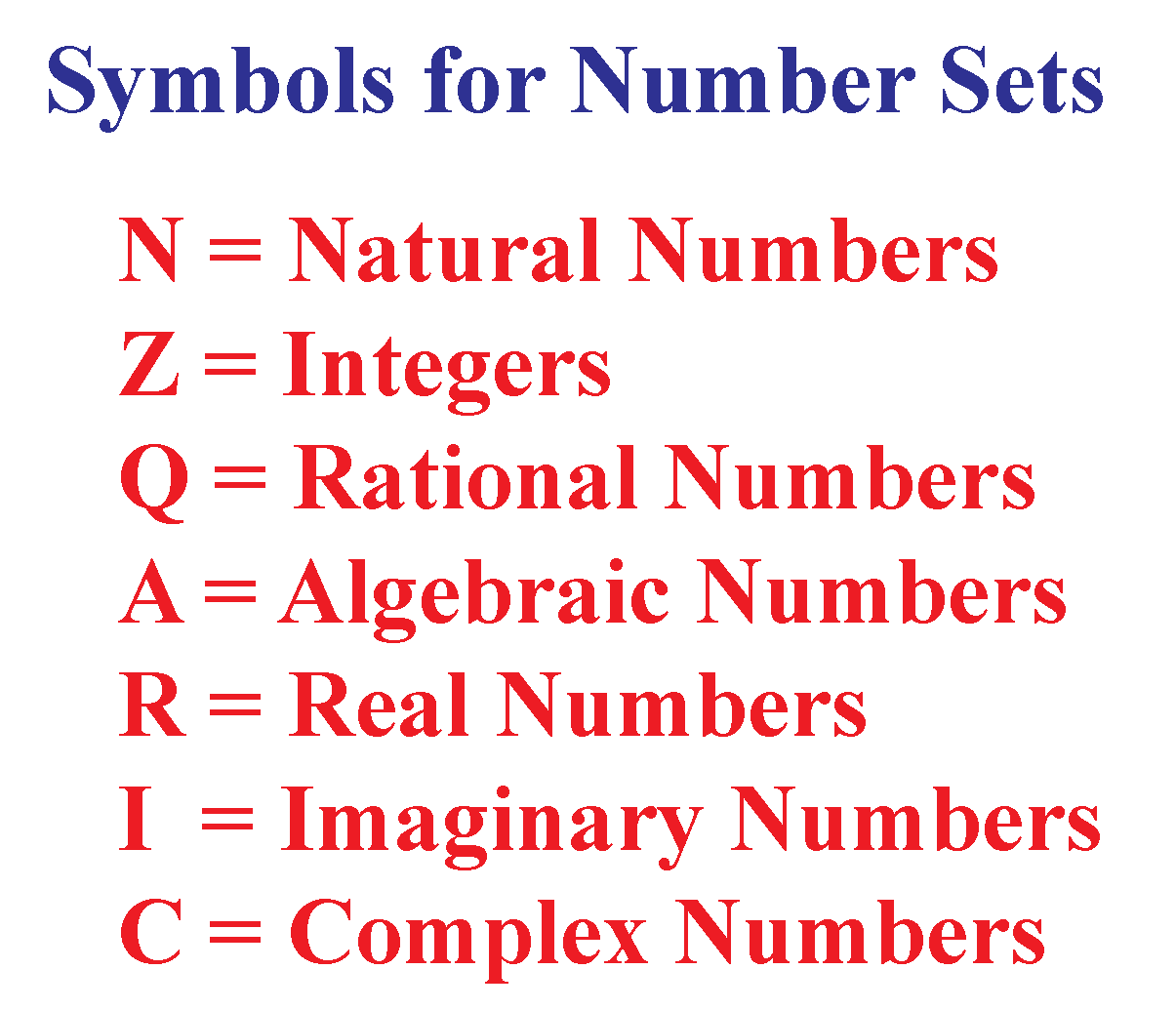Some of the below-given set of numbers are known to you.

$$N$$ = { 1, 2, 3, . . .} is a set of natural numbers.

$$W$$ = {0, 1, 2, 3, . . .} is a set of whole numbers.

$$I$$ = {..., -3, -2, -1, 0, 1, 2, ...} is a set of integers.

$$Q$$ is a set of rational numbers.

$$R$$ is a set of real numbers.

## What Is Set Builder Notation?

### Methods of Writing Set

There are two methods that can be used to represent a set.

(1) Listing method or roster method

In this method, we list down all the elements of a set inside curly brackets.

Each of the elements is written only once and is separated by commas.

For example, the set of letters in the word, "California" is written as A = {c, a, l, i, f, o, r, n}.

(2) Rule method or set builder form

In this method, we do not list the elements; instead, we will write the representative element using a variable followed by a vertical line or colon and write the general property of the same representative element.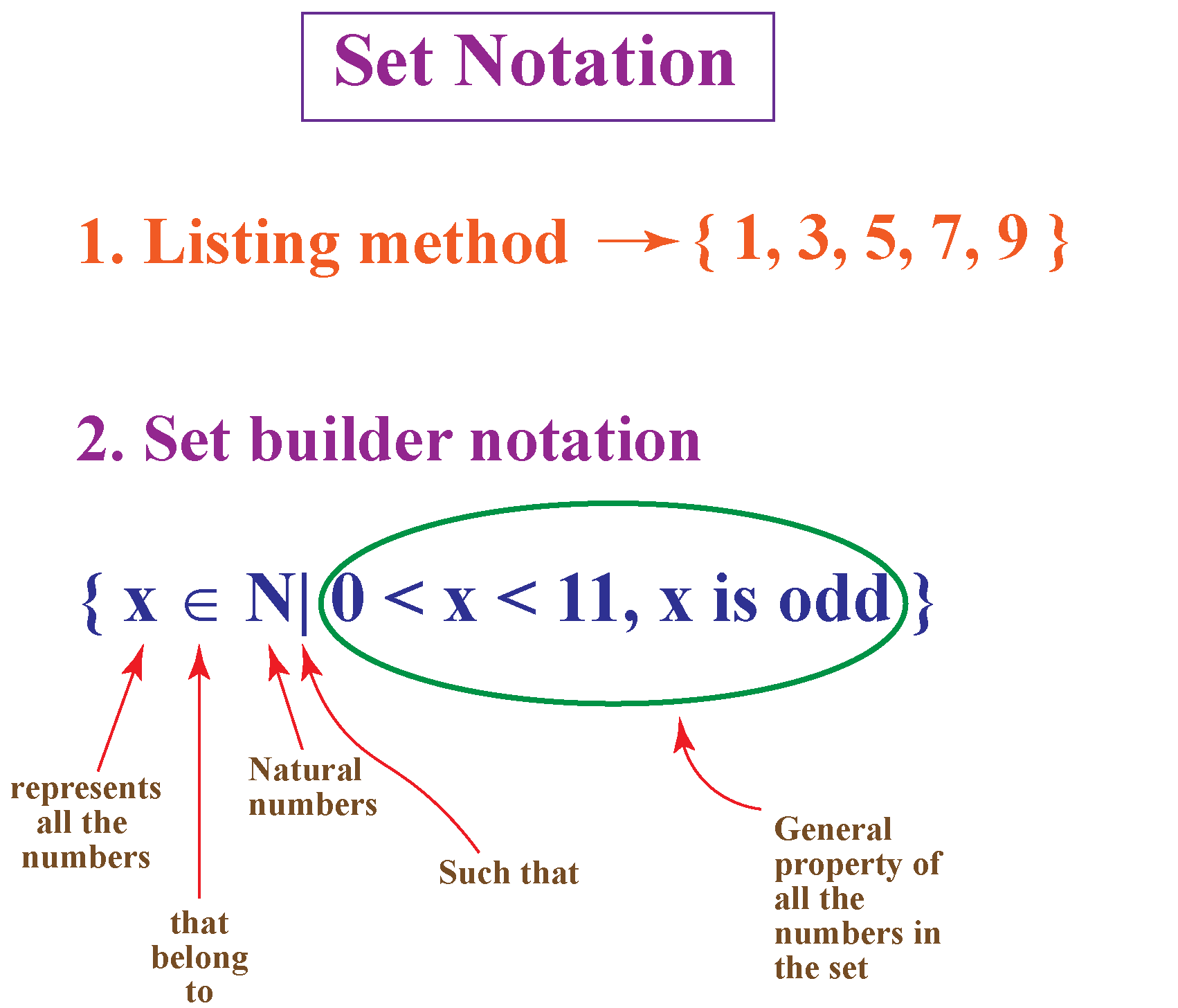Here are some set builder form examples.

Example:

$$A = \{x | x \in N, 5 < x < 10 \}$$ and is read as "set $$A$$ is the set of all ‘$$x$$’ such that ‘$$x$$’ is a natural number between 5 and 10."

The symbol $$\in$$ means “is an element of” and denotes membership of an element in a set.

Example:

$$B = \{ x | x \text { is an odd number between 11 and 20} \}$$ which means set $$B$$ contains all the odd numbers between 11 and 20.

By using the roster method, set $$B$$ can be written as $$B = \{11, 13, 15, 17, 19 \}$$

$$Q$$ is the set of rational numbers which can be written in set builder form as $\text Q = \{ \dfrac p q |\,p, q \,\in\, I, q \neq 0 \}$

The above is read as ‘$$Q$$’ is the set of all numbers in the form $$\dfrac q p$$ such that $$p$$ and $$q$$ are integers where $$q$$ is not equal to zero.’

In the following table, each set is written in both the methods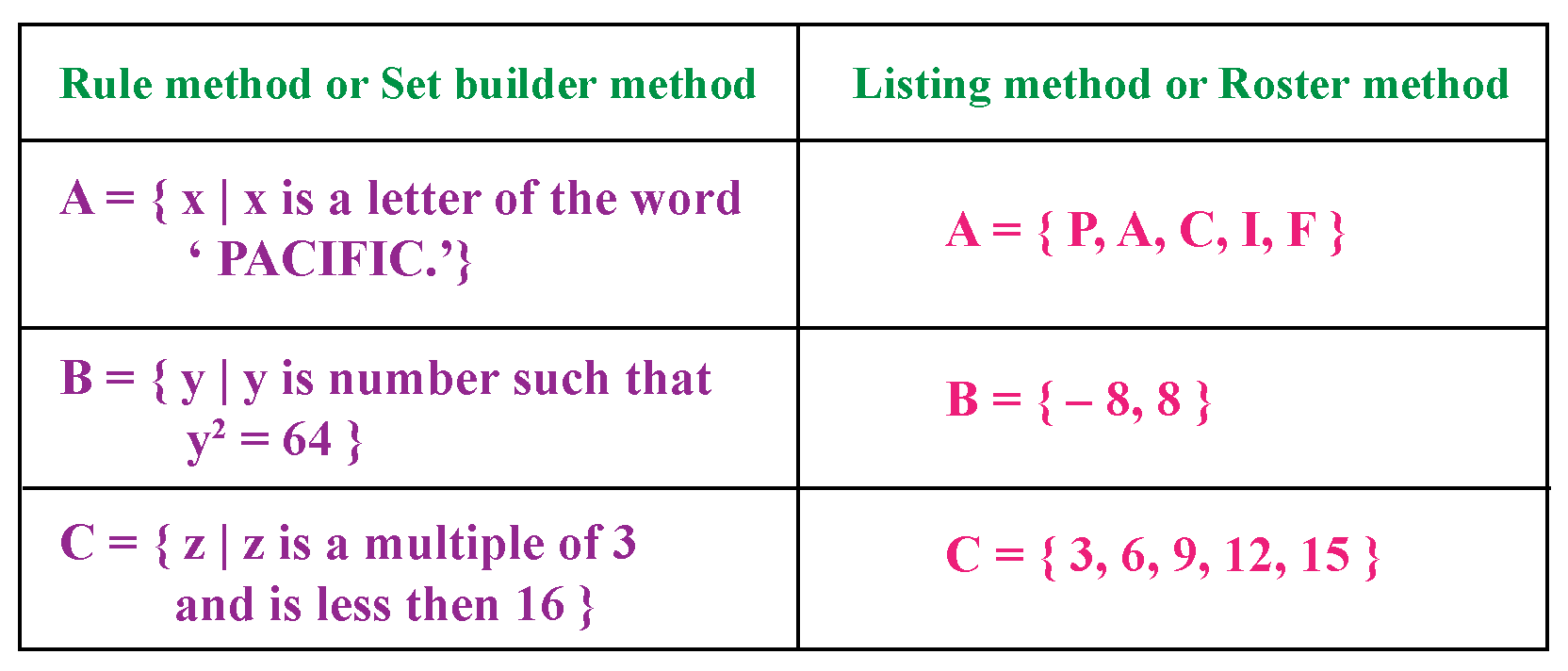## Set Builder Notation and Interval Notation

Interval notation is a way to define a set of numbers between a lower limit and an upper limit using end-point values.

The upper and lower limits may or may not be included in the set.

The end-point values are written between brackets or parentheses.

A square bracket denotes inclusion in the set, while a parenthesis denotes exclusion from the set.

For example,

(4,12]

The above interval notation denotes that this set includes all real numbers between 4 and 12

12 is included in the set while 4 is not a part of the set.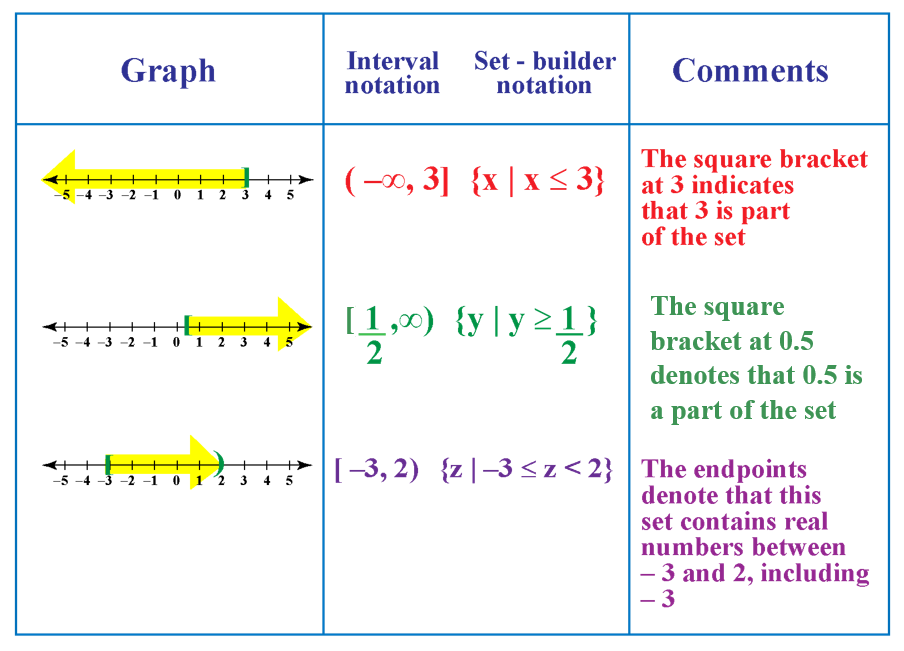Challenging Questions

1. Can you identify which of the following are equal sets and which are not? Justify your answer.

A = {$$x | 3x -1 = 2$$}
B = {$$x | x$$ is a natural number but $$x$$ is neither prime nor composite}
C = {$$x | x ∈ N, x < 4$$}

2. Write the following sets using the rule method.

(i) A = { 1, 4, 16, 25, 49, 64, 81, 100}

(ii) B = { 6, 12, 18, 24, 30, 36, 42, 48}

## Why Do We Use Set Builder Notation?

One might wonder why is there a need for such a complex notation!

What is the importance of set builder notation?If you have to list a set of integers between 1 and 8 inclusive, one can simply use roster notation to write {1, 2, 3, 4, 5, 6, 7, 8}.

But the problem arises when we have to list the real numbers in the same interval.

Using roster notation would not be practical.

{1, 1.1, 1.01, 1.001, 1.0001, ... ??? }

Using the set-builder notation would be better in this scenario.

Starting with all real numbers, we can limit them to the interval between 1 and 8 inclusive.

$$\{ x | x ≥ 1$$ and $$x ≤ 8 \}$$

It is quite convenient to use set builder notation to express other algebraic sets, such as:

$$\{ x | x = x^{2} \}$$

Set-builder notation comes in handy to write sets, especially for sets with an infinite number of elements.

Numbers such as integers, real numbers, and natural numbers can be expressed using set-builder notation.

A set with an interval or an equation can also be expressed using this method.

### Set Builder Notation for Domain and Range

Set builder notation is very useful for defining the domain and range of a function.

In its simplest form, the domain is the set of all the values that go into a function.

Example: For a function $$\text f(x) = \dfrac 2 {x -1}$$,

the domain would be all real numbers, except +1

This is because the function f$$(x)$$ would be undefined when $$x$$ = 1

Thus, the domain for the above function can be expressed as $$\{x ∈ R | x ≠ 1\}$$

## What Are the Different Symbols in Set Builder Notation?

• $$\in$$ means "is an element of"
• $$\not \in$$ means "is not an element of"
• $$N$$ represents natural numbers or all positive integers.
• $$W$$ represents whole numbers.
• $$Z$$ indicates integers.
• $$Q$$ represents rational numbers or any number that can be expressed as a fraction.
• $$R$$ represents real numbers or any number that isn't imaginary.

## Solved Examples

 Example 1

Can you write the given set in the set-builder form?

A = { 2, 4, 6, 8, 10, 12, 14}

Solution

$$\text A = \{x | x$$ is an even natural number less than 15 $$\}$$

 A = {x | x is an even natural number less than 15}
 Example 2

Help Cathy decode the given symbolic representations.

(i) 3 $$\in$$ Q

(ii) -2 $$\not \in$$ N

(iii) $$\text A = \{a | a$$ is an odd number $$\}$$

Solution

Q is the set of rational numbers and N is the set of natural numbers.

(i) 3 $$\in$$ Q means 3 belongs to a set of rational numbers.

(ii) -2 $$\not \in$$ N means -2 does not belong to a set of natural numbers.

(iii)$$\text A = \{a | a$$ is an odd number $$\}$$ means $$A$$ represents the set of $$a$$ elements and $$a$$ represents all the numbers that are odd.

 Example 3

Jamie was asked to remove the incorrect elements of set D.

He is unable to recognize the incorrect elements.

Help him rewrite the set D listing all the correct elements.

$$D = \{(x , y) | x, y ∈ W, xy = 9 \}$$

$$D = \{(9, 1), (-1, -9), (-3, -3), (3, 3) \}$$

Solution

$$W$$ represents the set of whole numbers.

Thus, set D cannot have negative numbers.

Set D can be corrected as:

$$D = \{(9, 1), (3, 3) \}$$

 $$\therefore$$ $$D = \{(9, 1), (3, 3) \}$$
 Example 4

Julia's teacher has asked her to express the set which includes all the natural numbers in the world using interval notation.

Can you help her find the answer?

Solution

The first natural number is 1 and hence, 1 would be included in the set.

There are infinite natural numbers; we can proceed with their listing as 1, 2, 3, 4, ....and so on.

The set containing all the natural numbers in the world can be expressed in interval notation as

$$[1,\infty)$$

 $$\therefore$$ $$N= [1,\infty)$$

## Interactive Questions

Here are a few activities for you to practice. Select/Type your answer and click the "Check Answer" button to see the result.

## Let's Summarize

The mini-lesson targeted the fascinating concept of set-builder notation. We also learned set-builder notation for writing domain and range, set builder notation for even numbers, and set builder form examples. The math journey around set builder notation starts with what a student already knows, and goes on to creatively crafting a fresh concept in the young minds. Done in a way that not only it is relatable and easy to grasp, but also will stay with them forever. Here lies the magic with Cuemath.

At Cuemath, our team of math experts is dedicated to making learning fun for our favorite readers, the students!

Through an interactive and engaging learning-teaching-learning approach, the teachers explore all angles of a topic.

Be it worksheets, online classes, doubt sessions, or any other form of relation, it’s the logical thinking and smart learning approach that we, at Cuemath, believe in.

## 1. How do you express intervals in set builder notation?

For the given set A = {..., -3, -2, -1, 0, 1, 2, 3, 4}

A = {x ∈ Z | x ≤ 4 }

## 2. How do you write inequalities in set builder notation?

{ x | x ∈ R, x ≥ 2 and x ≤ 6 }

This indicates that the set includes all the real numbers, between 2 and 6 inclusive.

More Important Topics
Numbers
Algebra
Geometry
Measurement
Money
Data
Trigonometry
Calculus
More Important Topics
Numbers
Algebra
Geometry
Measurement
Money
Data
Trigonometry
Calculus